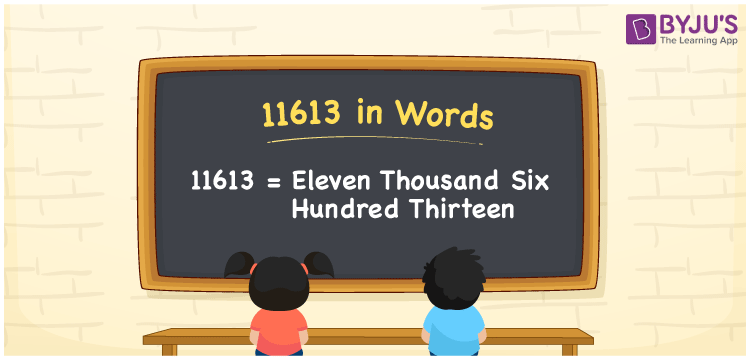# 11613 in words

11613 in words is written as Eleven Thousand Six Hundred and Thirteen. In 11613, the first 1 has a place value of ten thousand, the second 1 is in the place value of thousand, 6 is in the place value of hundred, next 1 is in the place value of ten and 3 is in the place value of ones. The article on Place Value gives more information. The number 11613 is used in expressions that relate to money, distance, social media views, and many more. For example, “The Employee number of my colleague is Eleven Thousand Six Hundred and Thirteen.”

 11613 in words Eleven Thousand Six Hundred and Thirteen Eleven Thousand Six Hundred and Thirteen in Numerical Form 11613

## 11613 in English Words## How to Write 11613 in Words?

We can convert 11613 to words using a place value chart. The number 11613 has 5 digits, so let’s make a chart that shows the place value up to 5 digits.

 Ten thousand Thousands Hundreds Tens Ones 1 1 6 1 3

Thus, we can write the expanded form as:

1 × Ten thousand + 1 × Thousand + 6 × Hundred + 1 × Ten + 3 × One

= 1 × 10000 + 1 × 1000 + 6 × 100 + 1 × 10 + 3 × 1

= 11613.

= Eleven Thousand Six Hundred and Thirteen.

11613 is the natural number that is succeeded by 11612 and preceded by 11614.

11613 in words – Eleven Thousand Six Hundred and Thirteen.

Is 11613 an odd number? – Yes.

Is 11613 an even number? – No.

Is 11613 a perfect square number? – No.

Is 11613 a perfect cube number? – No.

Is 11613 a prime number? – No.

Is 11613 a composite number? – Yes.

## Solved Example

1. Write the number 11613 in expanded form

Solution: 1 x 10000 + 1 x 1000 + 6 x 100 + 1 x 10 + 3 x 1

We can write 11613 = 10000 + 1000 + 600 + 10 + 3

= 1 x 10000 + 1 x 1000 + 6 x 100 + 1 x 10 + 3 x 1.

## Frequently Asked Questions on 11613 in words

Q1

### How to write the number 11613 in words?

11613 in words is written as Eleven Thousand Six Hundred and Thirteen.
Q2

### State whether True or False. 11613 is divisible by 3?

True. 11613 is divisible by 3.
Q3

### Is 11613 divisible by 10?

False. 11613 is not divisible by 10.
Test your Knowledge on 11613 in Words